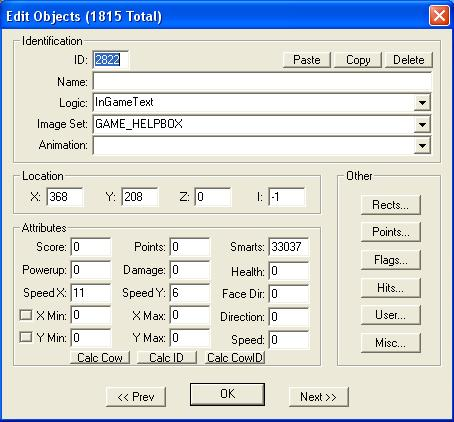CalcCowID

When a logic is placed into the map in Object Mode, two Edit Objects Dialog Box fieldz are filled in:• Location X: the number of pixels from the left edge (368 in the picture above)
• Location Y: the number of pixels from the top edge (208 in the picture above)
and many fieldz receive 'default' valuez (usually 0).

Certain Object logicz require the CalcCowID button exist, and be pressed before pressing the "OK" button ... such as Switchez.

Three Edit Objects Dialog Boxez are filled in by this action:

ID: ##### calculated value.
Speed X: 0 (replaced with X Tile coordinate after CalcCowID)
Speed Y: 0 (replaced with Y Tile coordinate after CalcCowID)
 Calculation (the easy way): 256X + Y = ID: ... but assume that both X and Y are unknown, so Calculation (the hard way): ({Location X:}/32, ignore remainder) = Speed X: ({Location Y:}/32, ignore remainder) = Speed Y: 256X + Y = ID:
Given: {Location X:} = 368, {Location Y:} = 208
Then: (368/32, ignore remainder) = 11 as the value for Speed X:
and: (208/32, ignore remainder) = 6 as the value for Speed Y:
Thus: (256 X 11) = 2816 + 6 = 2822 = ID:

Simple, right? So let's do another!

Given: {Location X:} = 814, {Location Y:} = 1135
Then: (814/32, ignore remainder) = 25 as the value for Speed X:
and: (1135/32, ignore remainder) = 35 as the value for Speed Y:
Thus: (256 X 25) = 6400 + 35 = 6435 = ID:

GooRoo's strong suggestion:
Do a CalcCowID on every Object you add into your Custom Level!

For one thing, all you then need to do to tell where you placed that Megaphone is click on its image to display the X and Y coordinates. Then you may easily enter its location into your Walkthrough component "Megaphonez.html" (you are writing a walkthrough, aren't you?). And if you want a SecretTeleportTrigger to open up a Red Warp to take a Grunt nearby, knowing where that Megaphone is located will make entering the necessary X and Y Tile coordinatez into that Object logic far easier! (And it makez it a lot easier for me to troubleshoot any problem the Designer reportz having with hir (combination of "his" and "her") Custom Level!